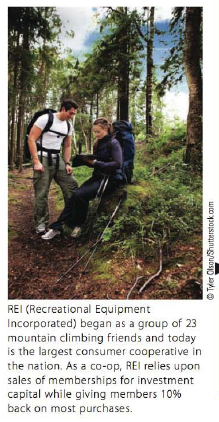Chapter 5.II, Problem 16TIE### Contemporary Mathematics for Busin...

8th Edition
Robert Brechner + 1 other
ISBN: 9781305585447

#### Solutions

Chapter
Section### Contemporary Mathematics for Busin...

8th Edition
Robert Brechner + 1 other
ISBN: 9781305585447
Textbook Problem

# REI (Recreational Equipment Incorporated) sells a 35 -liter backpack for $110 and an 18-liter backpack for$70. If a store sold 40 packs yesterday for a total of $3, 400, how many of each type backpack were sold? What were the dollar sales of each type?To determine To calculate: The number of each backpack sold and the amount received from the sale of each backpack, if REI (Recreational equipment incorporated) charges$110 for 35-liter backpack and $70 for 18-liter backpack. The store received$3,400 from the sale of 40 packs.

Explanation

Given Information:

REI (Recreational equipment incorporated) charges $110 for 35-liter backpack and$70 for 18-liter backpack. The store received $3,400 from the sale of 40 packs. Formula used: To solve the equation and prove the solution, follow the steps as mentioned below. Step 1: Understand the problem and write the facts of the equation. Step2: List the known and the unknows from the problem. Step3: Write the equation with the help of following table of key word,  Equal Sign Addition Subtraction Multiplication Division Parentheses Is And Less of Divide Times the quantity of Are Added to Less than Multiply Divided by Was Totals Smaller than Times Divided into Equals The sum of Minus Product of Quotient of Gives Plus Difference Multiplied by Ratio of Giving More than Decreased by Twice Leaves Larger than Reduced by Double Results in Increased by Take away Triple Produces Greater than Loss of At yields exceeds Fewer than @ Step4: Solve the equation by rearrange the unknown values to the left side and known values to the right side of the equal sign. Step5: Prove the solution by substituting the value obtained from step 4 in the equation formed in step 4 then check the left and right of the equation if, they are equal then the answer is correct. Calculation: Underline the keyword by using the table of of key word. The sum of price of each item multiply by its quantity equals total price,$3,400.

Suppose that X be the number of 35-liter backpack sold.

Consider the total backpack sold, 40.

So, the number of 18-liter backpack sold is, 40X.

Consider the charges for 35-liter backpack is $110 and for 18-liter backpack is$70.

Consider the amount received by store from the sale of 40 packs,

110X+70(40X)=\$3,400.

Now solve the equation

110X+70(40X)=3,400

Solve the parenthesis and combine the unknowns on left side,

110X+70(40X)=3,400110X+70(40)70(X)=3,400110X+280070X=3,40040X+2800=3,400

Arrange the known values to the right side and unknown to the left side by applying the opposite operation of addition, subtract 2800 from both sides,

40X

### Still sussing out bartleby?

Check out a sample textbook solution.

See a sample solution

#### The Solution to Your Study Problems

Bartleby provides explanations to thousands of textbook problems written by our experts, many with advanced degrees!

Get Started

#### Solve the equations in Exercises 126. (x3+1)x+1(x3+1)2x+1=0

Finite Mathematics and Applied Calculus (MindTap Course List)

#### In Exercises 73-80, find the indicated limits, if they exist. 78. limx4x43x2+12x4+x3+x2+x+1

Applied Calculus for the Managerial, Life, and Social Sciences: A Brief Approach

#### Find the 1000th derivative of f(x) = xex.

Single Variable Calculus: Early Transcendentals, Volume I

#### Which is the best graph of r = 1 − sin θ for 0 ≤ θ ≤ π?

Study Guide for Stewart's Single Variable Calculus: Early Transcendentals, 8th

#### The angle θ at the right has cosine:

Study Guide for Stewart's Multivariable Calculus, 8th